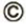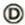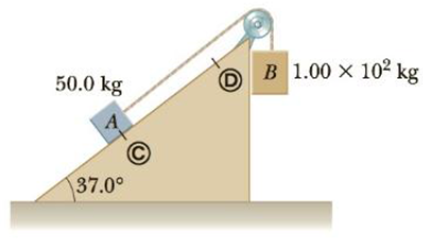Chapter 5, Problem 86AP

Chapter
Section
Textbook Problem

Two blocks, A and B (with mass 50.0 kg and 1.00 × 102 kg, respectively), are connected by a string, as shown in Figure P5.86. The pulley is frictionless and of negligible mass. The coefficient of kinetic friction between block A and the incline is μk = 0.250. Determine the change in the kinetic energy of block A as it moves fromto, a distance of 20.0 m up the incline (and block B drops downward a distance of 20.0 m) if the system starts from rest.Figure P5.86

To determine
The change in kinetic energy of block A as it moves from C to D.

Explanation

Section1:

To determine: The speed of the two blocks.

Answer: The speed of the two blocks is 12.53m/s .

Explanation:

Given Info:

The mass of block A is 50.0kg .

The mass of block B is 1.0×102kg .

The coefficient of kinetic friction between block A and incline is 0.250 .

The vertical displacement of the block B is 20.0m .

The normal force on the block A by incline is,

nA=(mAg)cos37.0°

• mA is the mass of block A
• g is the acceleration due to gravity

The friction force between block A and incline is,

fK=μknA=μk(mAgcos37.0°)

• μk is the coefficient of kinetic friction between block A and incline

The distance that the block A rises vertically is,

ΔhA=(20.0m)sin37.0°=12.0m

From the Work-Energy theorem,

Wnc=(KEf+PEg,f)(KEi+PEg,i)=ΔKE+ΔPEg

Therefore,

(μkmAgcos37.0°)s=[12(mA+mB)vf20]+(mAg(ΔhA)+mBg(ΔyB))

• vf is the final velocity of the block

On re-arranging,

Formula to calculate the final speed of the block is,

vf=2g[mB(ΔhB)mA(ΔhA)(μAmAcos37.0°)s]mA+mB

Substitute 9

Still sussing out bartleby?

Check out a sample textbook solution.

See a sample solution

The Solution to Your Study Problems

Bartleby provides explanations to thousands of textbook problems written by our experts, many with advanced degrees!

Get Started